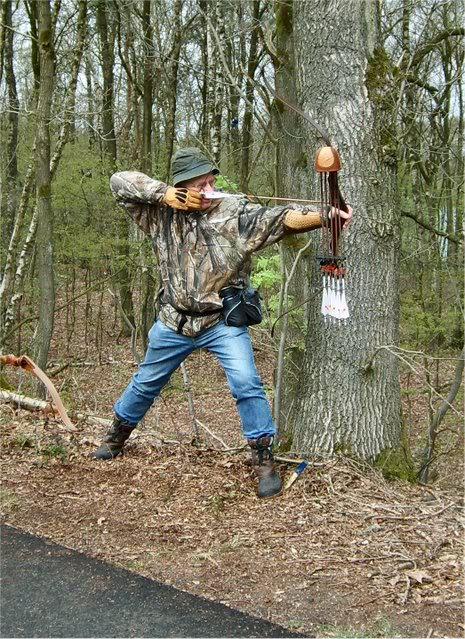# Don't shoot the animal, hunter!

Probability Level 5A sportsman's chance of shooting an animal at a distance $R(>A)$ is $\frac{A^{2}}{R^{2}}.$ He fires when $R=2A$ and if he misses, he reloads and fires when $R=3A,4A,5A\cdots$ and so on. If he misses at distance $1729A,$ the animals escapes.

If the probability that the animal escapes is $\frac{a}{b}$ where $a$ and $b$ are coprime integers, what is the value of $b-a?$

You can try my other Probability problems by clicking here

×